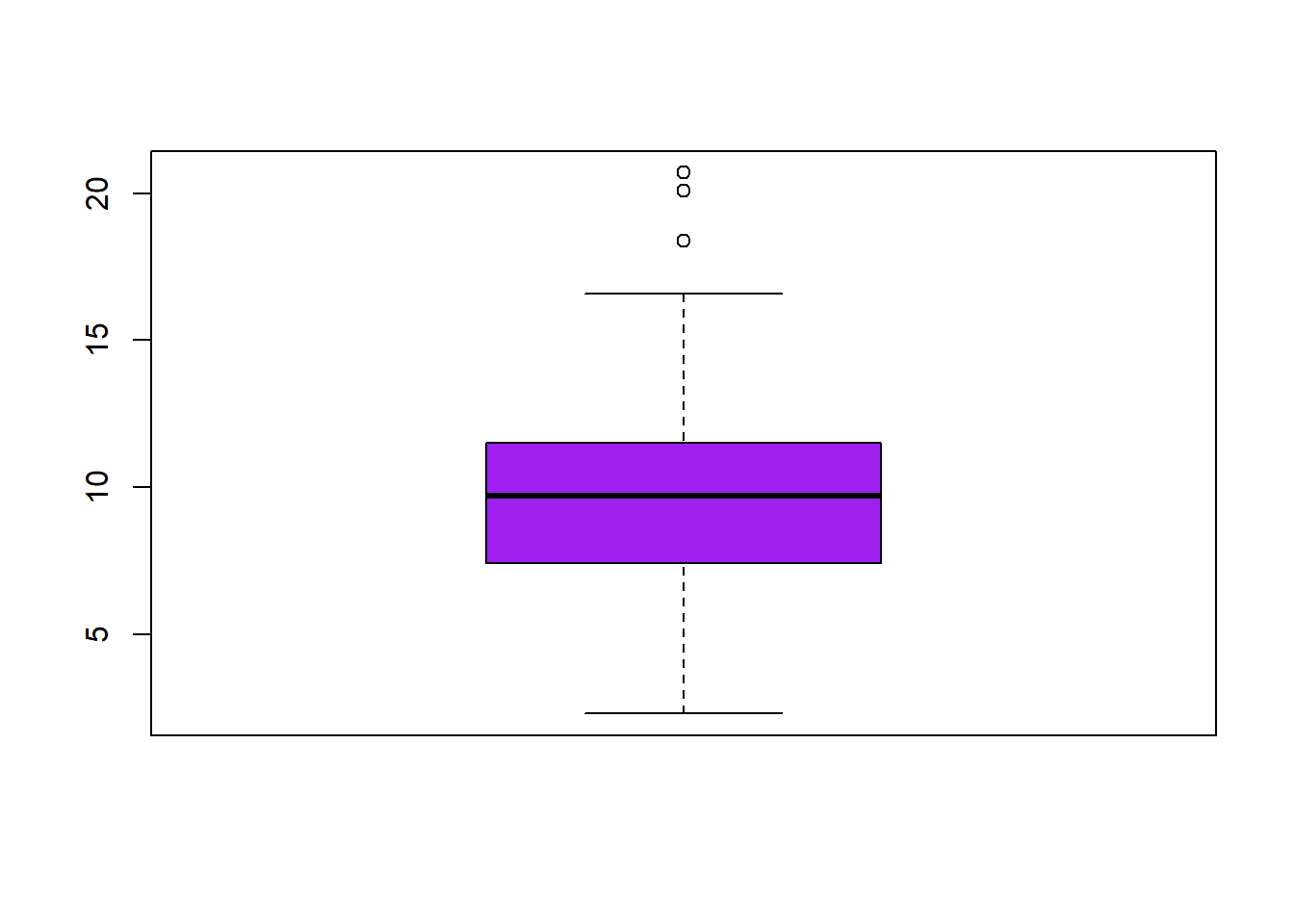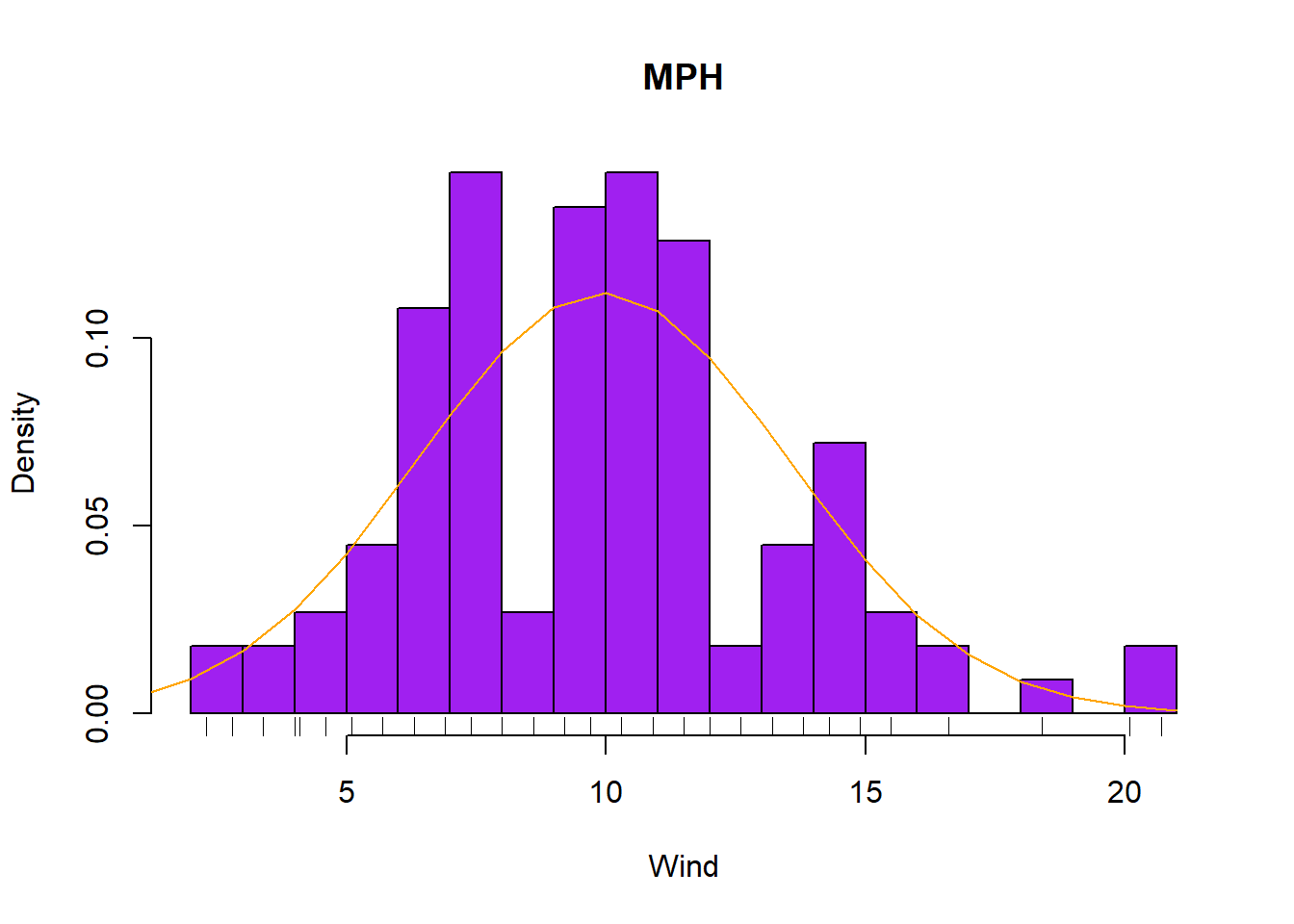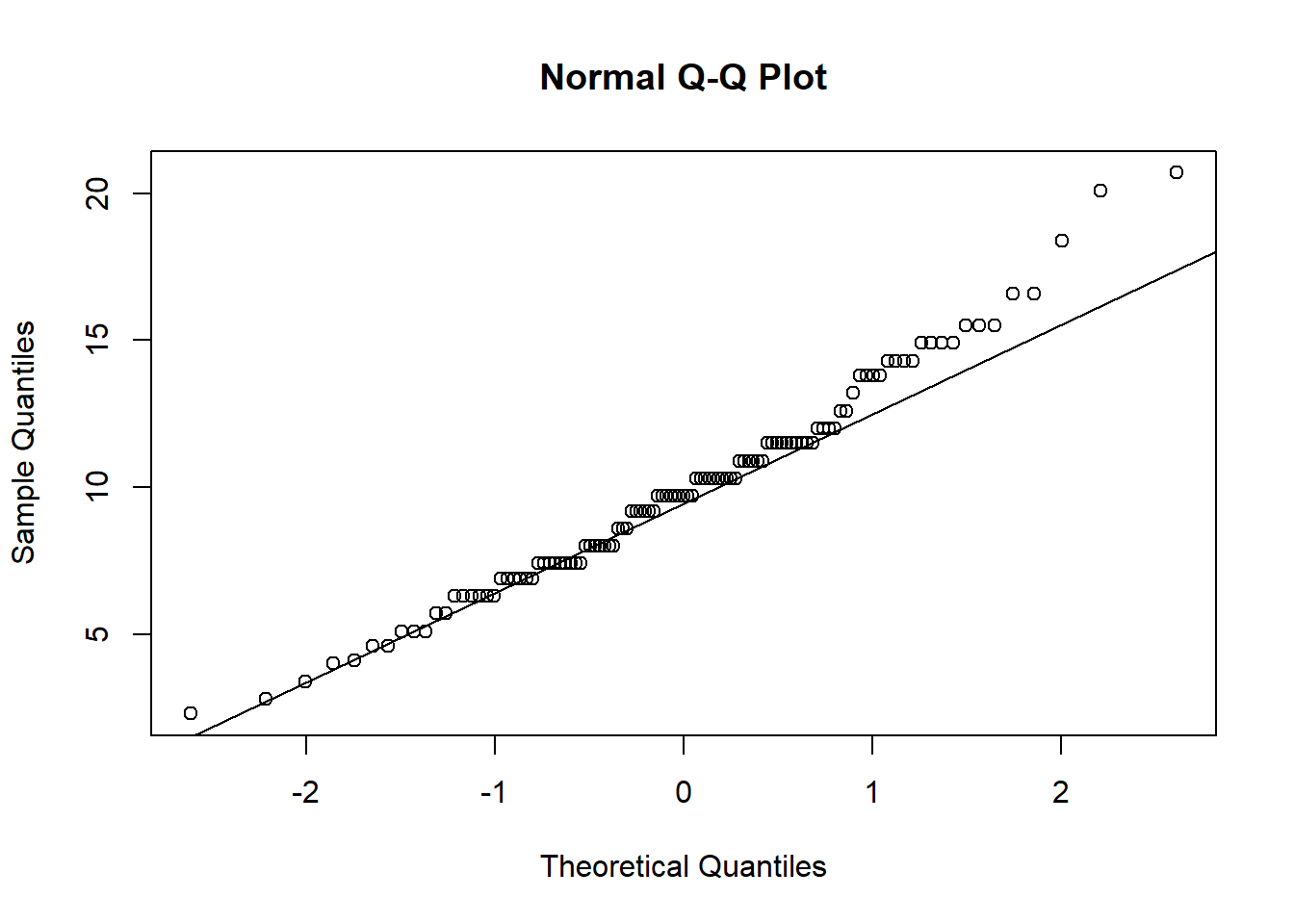##    Min. 1st Qu.  Median    Mean 3rd Qu.    Max.
##    2.30    7.40    9.70    9.94   11.50   20.70
## 'data.frame':    111 obs. of  6 variables:
##  $Ozone : int 41 36 12 18 23 19 8 16 11 14 ... ##$ Solar.R: int  190 118 149 313 299 99 19 256 290 274 ...
##  $Wind : num 7.4 8 12.6 11.5 8.6 13.8 20.1 9.7 9.2 10.9 ... ##$ Temp   : int  67 72 74 62 65 59 61 69 66 68 ...
##  $Month : int 5 5 5 5 5 5 5 5 5 5 ... ##$ Day    : int  1 2 3 4 7 8 9 12 13 14 ...

The mean is close to the median value so we assume skewness won’t be a concern. We have 153 observations of 6 variables of mostly type integer. Duplicates and null values were omitted.The boxplot shows that there are three outliers with a value greater than 18 mph, which occur in months 5(May) and 6(June). They are considered outliers since they have a value larger than 1.5 * the inner quartile range(IQR) above the upper quartile.

The median is slightly closer to the upper quartile indicating a negative skew.

Thankfully we do not have any values less than zero. Since we cannot have a negative MPH it would be strange to see, and indicate a data entry error.

Regression analysis does not respond well to outliers and a threshold of 17 would help in that case.The histogram with a density plot shows that the distribution peaks at 10 mph, and that the data is normally distributed. However, if we look at the Normal Q-Q plot we can see how the outliers lead to deviations from the theoretical line of a Gaussian distribution, and skewedness to the right where the imperical quintiles are larger than the theoretical quintiles making them heavier.